IF YOU CAN SEE THIS go to /sfa/Nuprl/Shared/Xindentation_hack_doc.html

### Recursive Functions

Unlike most type theory based logics, Nuprl's computational type theory admits computation by general recursion. This is possible because how expressions are to be executed is prior to (independent of) type formation, and admits untyped methods of computation on expressions. For detail on how this is done, see Recursion via the Y-combinator.
Consider the factorial function.

Def x! == if x=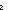0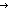1 else x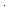(x-1)! fi  (recursive)

where ?=? is a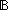-valued test of integer equality.

Here's an example of how it evaluates:

3! *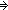3(3-1)! *32(3-1-1)! *321(3-1-1-1)! *3211 *6

Observe that execution (-1)! would never complete.

(-1)! *(-1)(-1-1)! *(-1)(-1-1)(-1-1-1)!, et cetera.

The argument that Thm*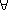x: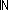x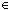is carried out by induction over.
The potential and convenience of general recursion is better demonstrated with the following variant of factorial that has a result only on non-negative even inputs.

Def x!== if x=01 else x(x-2)!fi  (recursive)

Thm*x:{x:| (x rem 2) = 0 }. x!Indeed, one can even type Kleene's paradigmatic unbounded search function,

Def mu(f) == if f(0)0 else 1+mu(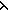x.f(1+x)) fi  (recursive)

Thm* mu{f:(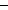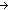)|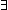x:f(x) }Thm*f:{f:()|x:f(x) }. f(mu(f))
Thm*f:{f:()|x:f(x) }, i:i<mu(f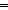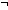f(i)

One can also define type-valued functions and predicates by general recursion on the domain. Here is a definition of n-tuples defined by iterating cartesian product. We include a degenerate singleton case for 0.
Note: this is not a recursive type, but a recursive definition of a family of types. See Recursive Types.

Def A^n == if n=0Unit ; n=1A else A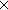(A^(n-1)) fi  (recursive)

Thm*A:Type, n:. (A^nType

Now let us compute:

A^0 *Unit
A^1 *A
A^2 *AA
A^3 *AAA

Here's a predicate on n-tuples to the effect that a tuple includes a value satisfying some property of the element type (see Propositions).

Defu in XA^nP(u)
Def == n = 1& P(X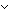n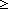2 & (X/a,restP(a(u in restA^(n-1). P(u)))
Def (recursive)

Thm*A:Type, P:(AProp), n:X:(A^n). (u in XA^nP(u))Prop

Example of use:
Thm*i in <1,(-1),0>: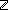^3. i<0

(March 2001 - sfa )